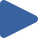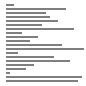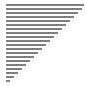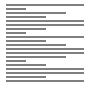# Shell Sort

## Animation, code, analysis, and discussion of shell sort on 4 initial conditions.

How to use: Press "Play all", or choose thebutton.

 Play All Play animationRandomPlay animationNearly SortedPlay animationReversedPlay animationFew Unique### ALGORITHM

``````h = 1
while h < n, h = 3*h + 1
while h > 0,
h = h / 3
for k = 1:h, insertion sort a[k:h:n]
→ invariant: each h-sub-array is sorted
end
``````

### DISCUSSION

The worse-case time complexity of shell sort depends on the increment sequence. For the increments 1 4 13 40 121…, which is what is used here, the time complexity is O(n3/2). For other increments, time complexity is known to be O(n4/3) and even O(n·lg2(n)). Neither tight upper bounds on time complexity nor the best increment sequence are known.

Because shell sort is based on insertion sort, shell sort inherits insertion sort’s adaptive properties. The adapation is not as dramatic because shell sort requires one pass through the data for each increment, but it is significant. For the increment sequence shown above, there are log3(n) increments, so the time complexity for nearly sorted data is O(n·log3(n)).

Because of its low overhead, relatively simple implementation, adaptive properties, and sub-quadratic time complexity, shell sort may be a viable alternative to the O(n·lg(n)) sorting algorithms for some applications when the data to be sorted is not very large.

### KEY

• Black values are sorted.
• Gray values are unsorted.
• Dark gray values show the current sub-array that is being sorted using insertion sort.
• A red triangle marks the algorithm position.

### PROPERTIES

• Not stable
• O(1) extra space
• O(n3/2) time as shown (see below)
• Adaptive: O(n·lg(n)) time when nearly sorted